×

Search anything:

# U-Net architecture

#### Machine Learning (ML)In this article, we have explained U-Net architecture along with other key ideas like Downsampling and Upsampling path with features and applications of U-Net.

1. Introduction to U-Net
2. U-Net Architecture
• DOWNSAMPLING PATH
• UPSAMPLING PATH
3. Training of U-Net
4. Some features of U-Net
5. Applications of U-Net

# Introduction to U-Net

In this article, we will be specifically discussing about the architecture of U-Net model.U-Net is an architecture for semantic segmentation, it made a huge impact on the biomedical sector as it helped in thorough image segmentation. It was developed in the year 2015, by Olaf Ronneburger, Philip Fischer and Thomas Brox at University of Freiburg ,Germany.

The traditional methods used the sliding window technique i.e each pixel was classified individually(also known as convolution) which was quite time taking.U-Net improved the working of convolutional neural network significantly, infact it wouldn't be wrong to say that U-Net was the best at the time and is still the state-of-art and is most commonly used for semantic segmentation tasks.It requires small number of training examples unlike the traditional convolutional networks that used thousands of annotated training samples.

# U-Net Architecture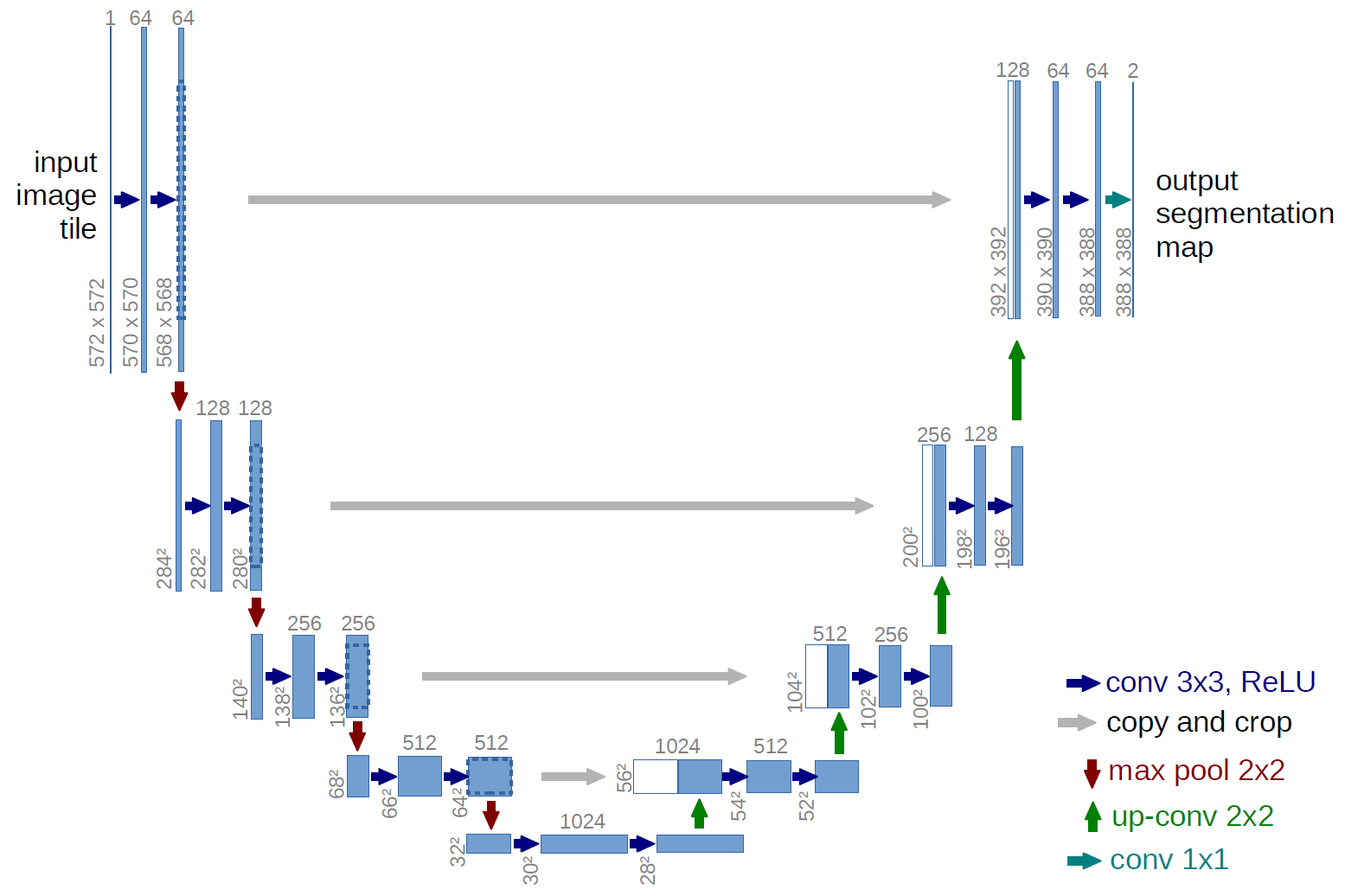As for the architecture,the U-Net model is in the shape of "U" as its name suggests;Consists of 2 paths namely : Downsampling path and Upsampling path.

## DOWNSAMPLING PATH

The downsampling path is mainly for the feature extractions.

In the downsampling path there are 4 convolution blocks, where each block has 2 convolution layers,that is of 3x3 padding followed by the ReLU (Rectified Linear Unit), 2x2 Max Pooling operator with 2 strides.

Number of filters are doubled with each consecutive convolution blocks.Initially there are 64 filters.Each filter helps in increase of depth.
The 5th convolution block does not have any max pooling operator, it just connects to the upsampling path.

## UPSAMPLING PATH

The upsampling path is for localization of objects.
In the upsampling path , there are 4 convolution blocks of 2 convolution layer of 2x2 . It is symmetric to that of the downsampling path .Each convolution increases resolution and decreases depth.Number of feature channel keep getting halved.The concolution layer is followed by 3x3 filters with ReLU activation.Final convolution layer is of 1x1 which helps in precise localization by mapping features to the appropriate classes.

Note: -The resolution of Input is better than that of resolution of Output.
-ReLU helps in converting all negative inputs to 0.
-Max pooling Operators simply take the max input value in the given stride.

# Training of U-Net

The network is trained by input images implemented with the stochiastic gradient descent. The stochiastic gradient descent optimizes by updating weights i.e subtracting the learning rate of its gradient from the current weight. The weights generated are important as they give more importance to certain pixels that highlight the image or help in segmentation.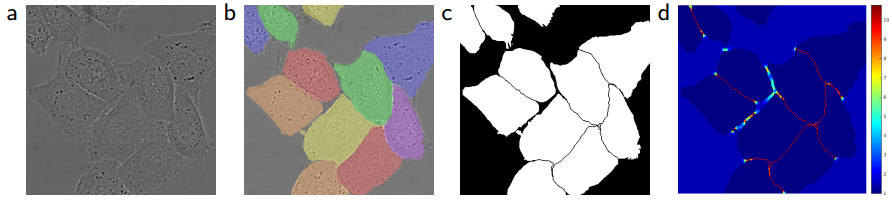As you can see in the above image , the borders of cells are given heigher weights in (d) part and you can clearly see the cell lining.
Softmax is an activation function and is formulated as: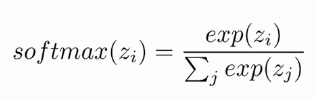On the output image this softmax is applied along with the cross entropy loss function.The softmax formula gives the probability distribution of the model by taking into consideration activation in feature channel at a certain position.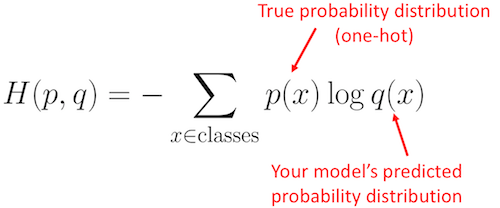In the above formula q(x) is replaced by softmax function and p(x) is replaced by weight at x;

Implementation of U-Net using pytorch:

``````import torch
import torch.nn as nn
import torchvision.transforms.functional as TF

class DoubleConv(nn.Module):
def __init__(self, in_channels, out_channels):
super(DoubleConv, self).__init__()
self.conv = nn.Sequential(
nn.Conv2d(in_channels, out_channels, 3, 1, 1, bias=False),
nn.BatchNorm2d(out_channels),
nn.ReLU(inplace=True),
nn.Conv2d(out_channels, out_channels, 3, 1, 1, bias=False),
nn.BatchNorm2d(out_channels),
nn.ReLU(inplace=True),
)

def forward(self, x):
return self.conv(x)

class UNET(nn.Module):
def __init__(
self, in_channels=3, out_channels=1, features=[64, 128, 256, 512],
):
super(UNET, self).__init__()
self.ups = nn.ModuleList()
self.downs = nn.ModuleList()
self.pool = nn.MaxPool2d(kernel_size=2, stride=2)

# Down part of UNET
for feature in features:
self.downs.append(DoubleConv(in_channels, feature))
in_channels = feature

# Up part of UNET
for feature in reversed(features):
self.ups.append(
nn.ConvTranspose2d(
feature*2, feature, kernel_size=2, stride=2,
)
)
self.ups.append(DoubleConv(feature*2, feature))

self.bottleneck = DoubleConv(features[-1], features[-1]*2)
self.final_conv = nn.Conv2d(features, out_channels, kernel_size=1)

def forward(self, x):
skip_connections = []

for down in self.downs:
x = down(x)
skip_connections.append(x)
x = self.pool(x)

x = self.bottleneck(x)
skip_connections = skip_connections[::-1]

for idx in range(0, len(self.ups), 2):
x = self.ups[idx](x)
skip_connection = skip_connections[idx//2]

if x.shape != skip_connection.shape:
x = TF.resize(x, size=skip_connection.shape[2:])

concat_skip = torch.cat((skip_connection, x), dim=1)
x = self.ups[idx+1](concat_skip)

return self.final_conv(x)

def test():
x = torch.randn((3, 1, 161, 161))
model = UNET(in_channels=1, out_channels=1)
preds = model(x)
assert preds.shape == x.shape

if __name__ == "__main__":
test()
``````

# Some features of U-Net

• In the U-Net architecture the INPUT given is always greater than OUTPUT generated because no padding was present in every convolution layer. Hence ,there are some missing data,which is filled with the technique of mirroring.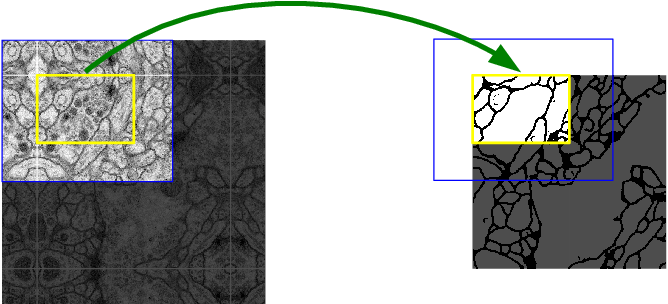]
• U-net also makes use of excessive data augmentation techniques by applying elastic deformations. Data augmentation is used to teach the network the desired invariance and robustness properties.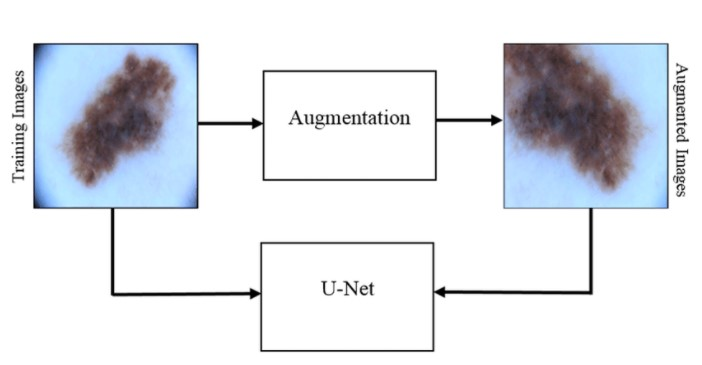]
• Touching objects of the same class label were made to diffrentiate based on weighted loss,where the seperating background labels between touching objects obtain a large weight in loss function.

# Applications of U-Net

• Use of Image Segmentation can be done in medical fields, self driving cars , machine learning etc , hence when some changes are made in the unet architecture these applications can be easily done.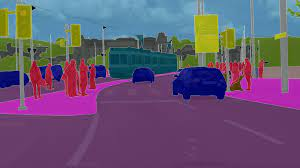• High resolution satellites also use such networks in order to better understand geological features .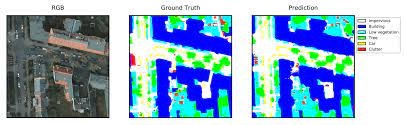• Unet can further be used for pose estimation, which can further help to understand the normalities/abnormalities of muscle positioning, bone structure and there movements.Pose estimation is widely used in games to copy human like movements.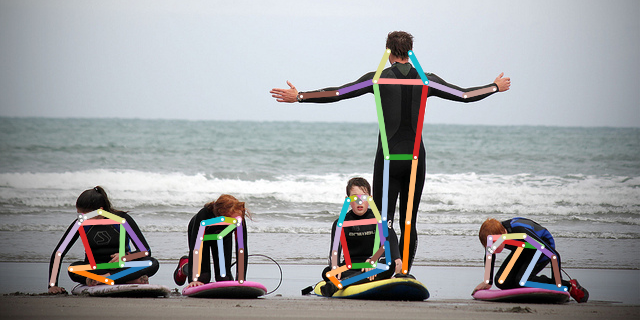FAQ's:

1. What is U-Net?
Soln:U-net is an image segmentation technique developed primarily for image segmentation tasks. These traits provide U-net with a high utility within the medical imaging community and have resulted in extensive adoption of U-net as the primary tool for segmentation tasks in medical imaging

Soln: U-Net is used when limited training samples is given and image segmentation has to be performed. U-net can also be used when low computation power is available.

3. What is data augmentation?
Soln:Data augmentation involves essential use of techniques that make multiple images by using elastic deformations on a few training sample.

Conclusion:

U-Net architecture can be used when the training samples are limited to achieve the best results. U-Net also provides precise localization when it comes to image segmentation. U-net also shows good performance on low computing power and hence is the reason that U-net architecture can be applied to many more tasks.

With this article at OpenGenus, you must have the complete idea of U-Net model.

#### Anubhav Tewari

Anubhav Tewari has been a Machine Learning Developer, Intern at OpenGenus.